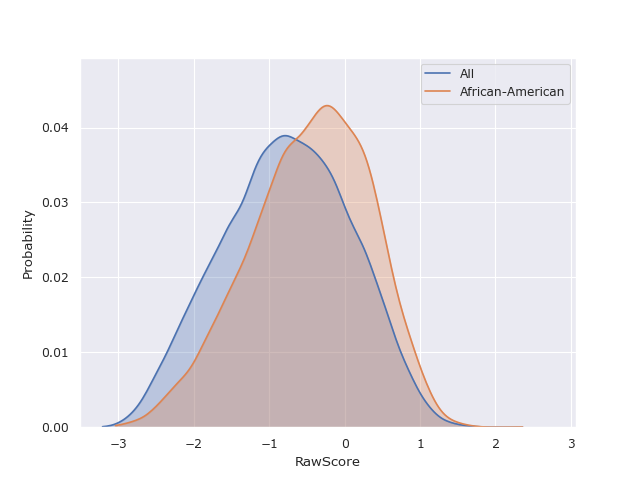# Overview#

In most supervised learning problems, a model is trained on a set of features $$X$$, to predict or estimate a target variable $$Y$$. The resulting prediction of a trained model is denoted by $$R$$. Additionally, we define $$A$$, a subset of $$X$$, which corresponds to legally protected attributes.

$$\underbrace{\text{Title}\quad \overbrace{\text{Gender}\quad \text{Ethnicity}}^{A}\quad \text{Legal Status}}_{X}\quad \overbrace{\text{Raw Score}}^{Y}\quad \overbrace{\text{Predicted Score}}^{R}$$ 1

There are multiple definitions of fairness in literature, and while many can be contentious, results are often considered fair if they are independent of legally protected characteristics such as age, gender, and ethnicity 2.

$P(R \mid A) = P(R)$

In practice, most legally protected attributes tend to be categorical, therefore for the sake of simplicity we model protected or sensitive variables as discrete random variables, which gives us the following for independence.

$P(R \mid A = a) = P(R)\quad \forall a \in A$
$\text{or}$
$P(R \mid A = a) = P(R \mid A = b)\quad \forall a,b \in A$

While Barocas et al. 2 proposes 2 alternative fairness or non-discrimination criteria, separation and sufficiency, which are applicable to a range of problems, we have chosen to work with independence because of its generality 3. Working with this abstract definition of fairness, we quantify the bias of a variable $$T$$ in a group $$a$$, as the statistical distance between the the probability distributions of $$P(T \mid A = a)$$ and $$P(T)$$.Using this definition, we can carry out hypothesis tests to measure the significance of an observed bias in a variable in a sensitive subgroup. The advantage of using this method is that the target variable can be continuous, categorical or binary, depending on the metric or test used.

FairLens consists of a range of metrics and tests for statistical similarity and correlation, along with Monte Carlo methods for hypothesis testing, which can be used to assess fairness in the aformentioned way. The fairness of a variable, with respect to sensitive attributes, is assessed by measuring the bias of the variable in each possible sensitive subgroup and taking the weighted average.

In practice, $$R$$ can be substituted with any column in the data. For instance, if $$R$$ is the target column $$Y$$ in a dataset, then using the above methods will yield results indicating inherent biases present in the dataset. This can be useful for mitigating bias in the data itself. On the other hand, using the predictions as $$R$$ will indicate algorithmic bias.